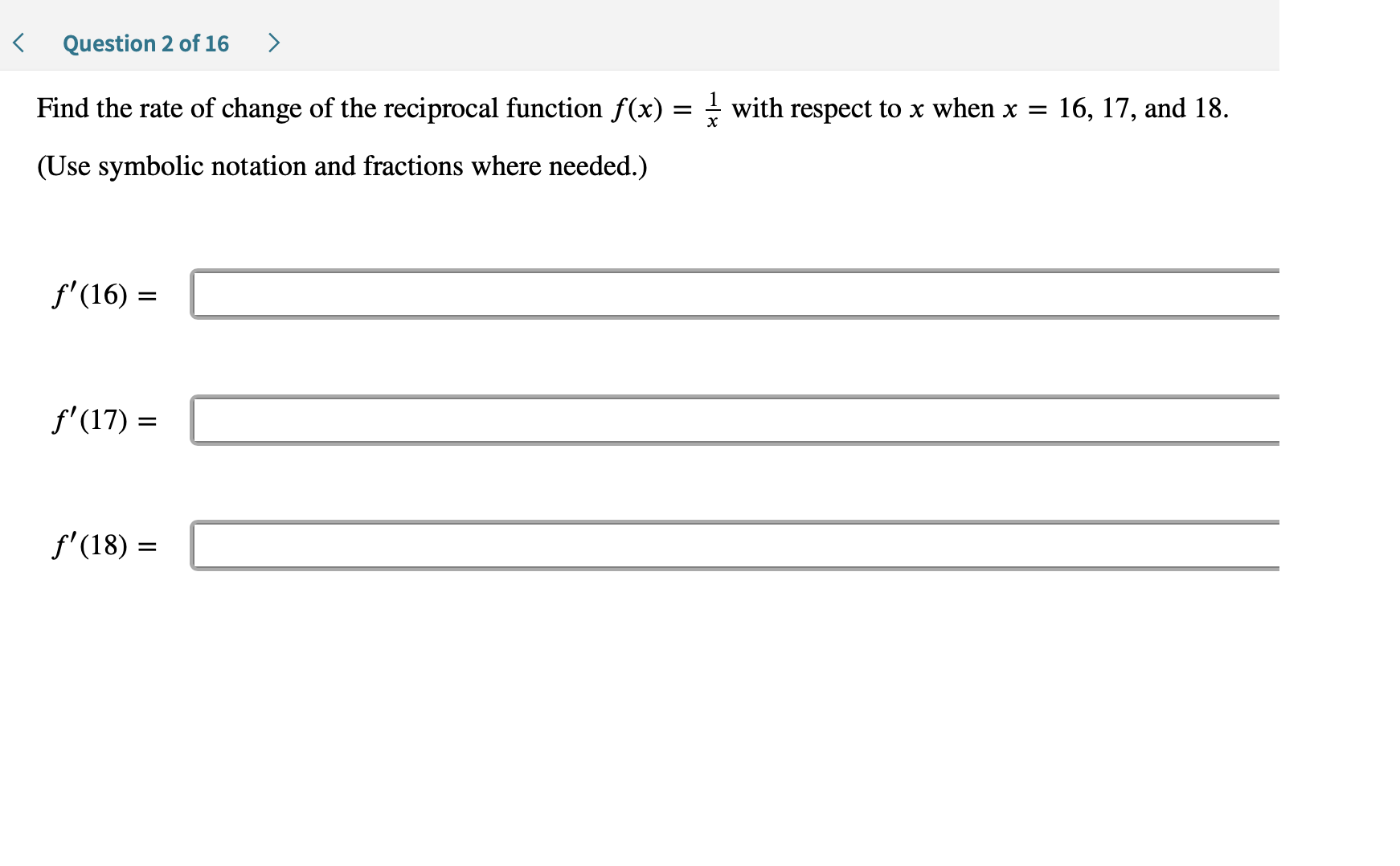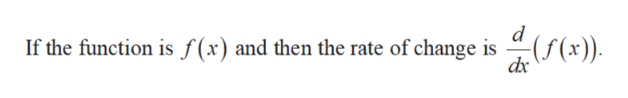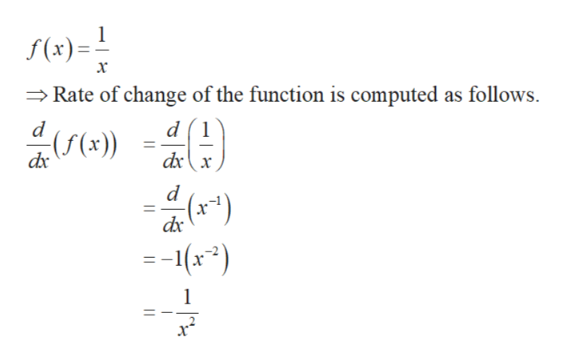# >Question 2 of 16Find the rate of change of the reciprocal function f(x) = - with respect to x when x =16, 17, and 18.(Use symbolic notation and fractions where needed.)f'(16)f'(17)f'(18)

Questionhelp_outlineImage Transcriptionclose> Question 2 of 16 Find the rate of change of the reciprocal function f(x) = - with respect to x when x = 16, 17, and 18. (Use symbolic notation and fractions where needed.) f'(16) f'(17) f'(18) fullscreen
check_circle

Step 1

Recall the following fact.help_outlineImage Transcriptionclosed If the function is f(x) and then the rate of change is dx fullscreen
Step 2

Find the rate of change of the given r...help_outlineImage TranscriptioncloseS(x)=! Rate of change of the function is computed as follows х d (1 ((x)) dx d -16x2) 1 fullscreen

### Want to see the full answer?

See Solution

#### Want to see this answer and more?

Solutions are written by subject experts who are available 24/7. Questions are typically answered within 1 hour.*

See Solution
*Response times may vary by subject and question.
Tagged in

### Derivative